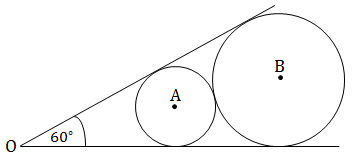# Nice to meet you

Geometry Level 3

(Refer to diagram below) Two lines intersect at $O$ at an angle of $60^\circ$. Two circles are drawn such that they are tangent to each other and the two lines. $A$ and $B$ are the centers of the smaller and larger, respectively. If the radius of the smaller circle is $15$, what is the length of $OB$?×